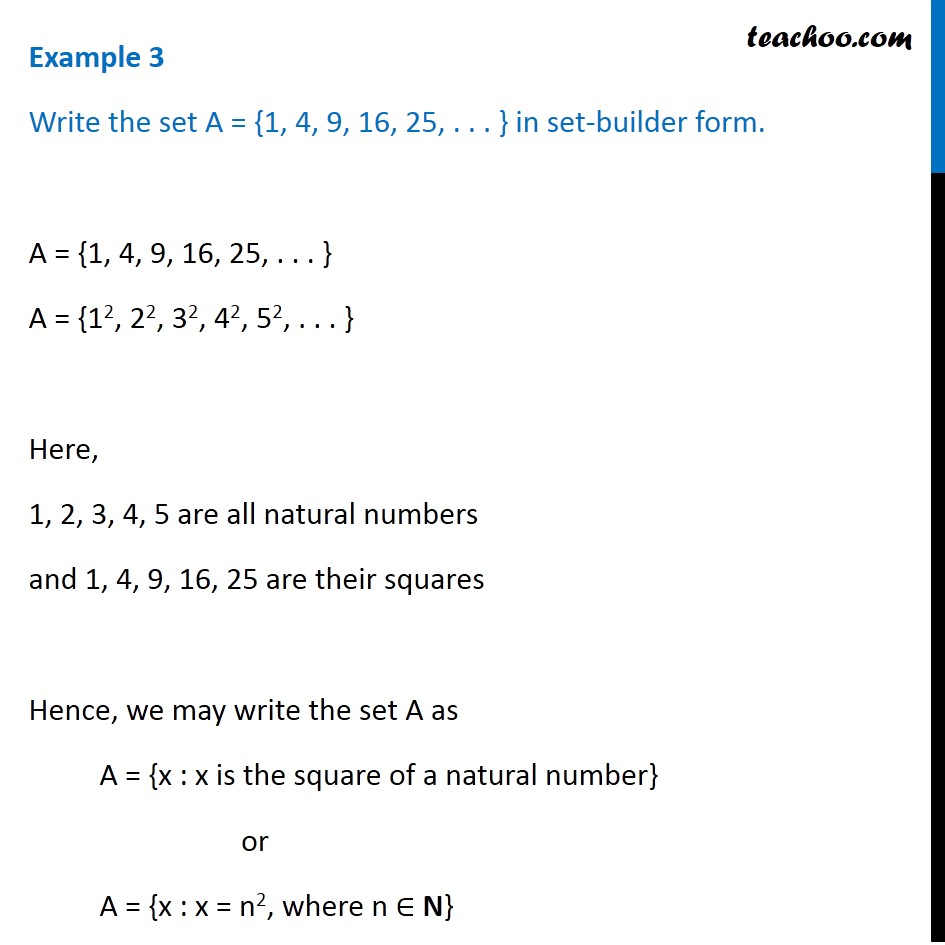1. Chapter 1 Class 11 Sets (Term 1)
2. Serial order wise
3. Examples

Transcript

Example 3 Write the set A = {1, 4, 9, 16, 25, . . . } in set-builder form. A = {1, 4, 9, 16, 25, . . . } A = {12, 22, 32, 42, 52, . . . } Here, 1, 2, 3, 4, 5 are all natural numbers and 1, 4, 9, 16, 25 are their squares Hence, we may write the set A as A = {x : x is the square of a natural number} or A = {x : x = n2, where n ∈ N}

Examples

Chapter 1 Class 11 Sets (Term 1)
Serial order wise